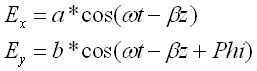# Antenna Polarization

The polarization of an antenna is the polarization of the wave radiated by the antenna. At a given position, the polarization describes the orientation of the electric field.

Suppose an electromagnetic wave, radiated by an antenna, has an electric field E ( a vector) with two components: E x and E y.We are going to explore the figure traced by the tip of vector E at a given position along the z axis as the time changes.

Let us assume that the components E x and E y of electric field E are given bywhere a is the amplitude of component E x and b is the amplitude of component E . Phi is the difference of phase between the two components.

Tutorial using an applet

Press the button below "press here to start". The left is for controlling parameters a, b and Phi and to start or stop the animation. Time t in the expressions giving the electric field is changed by steps or continuously. The electric field vector E changes in magnitude and direction. The extremity of the vector field E describes a curve: This curve defines the polarization.

Your browser is completely ignoring the <APPLET> tag!

## 1 - Linear polarization

Use the scrollbar to set Phi to 0. Set a = 1 and b = 1. (these values might be set already). Press the button "START/STOP animation". The trace of the field vector E should be linear of the hence this is linear polarization. Change a and b and observe the angle of the segment changing.
The condition for linear polarization is that Phi should take values such as : Phi = n*Pi, where n is an integer. Use different values for Phi = n*Pi and check that the polarization is linear.

## 2 - Circular polarization

Set a = b = 1 and Phi to 0.5Pi. Press the button "START/STOP animation". The trace is now a circle.
The conditions for circular polarization is that Phi should take values such as : Phi = (n+1)Pi/2. Example: Phi = Pi/2 , 3Pi/2, -Pi/2 ... and a = b. Take different values for Phi and a and b according the stated conditions and check that the polarization is circular.

## 3 - Elliptical polarization

Set a = b = 1 and Phi to any value other than 0, Pi, 0.5Pi, say 0.7Pi for example. Press the button "START/STOP animation". The trace is now an ellipse.
The conditions for elliptical polarization is that Phi should take values such as : Phi = (n+1)Pi/2. Example: Phi = Pi/2 , 3Pi/2, -Pi/2... Take different values for Phi according the stated conditions and check that the polarization is an ellipse if a is NOT equal to b.

More on antennas antennas and parabolic reflectors.